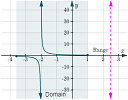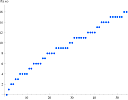Search IntMath
Close

# IntMath Newsletter: Domain, range, Azure, Riemann

By Murray Bourne, 11 May 2017

11 May 2017

1. New applet: Domain and range exploration
2. Resource: Azure Machine Learning Studio
3. Math in the news: Riemann hypothesis
4. Math movie: Is math discovered or invented?
5. Math puzzles: Bees
6. Final thought: Bees

## 1. New applet: Domain and range explorationThis interactive applet allows you to explore the concepts of domain and range for several different mathematical functions. Here's the applet: Domain and range exploration And here's some background to it: New applet: Domain and range investigation

## 2. Resource: Azure Machine Learning StudioFor those of you wondering what future jobs will involve, here's a resource that can give you some insights. Microsoft Azure Machine Learning Studio is a collaborative, drag-and-drop tool you can use to build, test, and deploy predictive analytics solutions on your data. It integrates with Python and R - software that math students should learn. There's a free option to try it out.

## 3. Math in the news: Riemann hypothesisQuestion: How many primes are there less than one million? Answer: 78,498 Question: Who cares? Answer: All of us, every time we log in to a bank account, or visit any secure Web site (and if we want to earn a million dollars).

Cryptography (the science of secret security codes) depends heavily on large primes, and the distribution of those primes is an important consideration for better security.

Mathematicians have tried to approximate the number of primes less than a given large number, and in 1859 the German mathematician Bernhard Riemann conjectured that it was related to the solutions of the Riemann zeta function, ζ(s). This appears to work (and it has been used ever since), but no one has ever proved it.

There's a $1 million prize waiting for anyone who does manage to solve it. Dorje Brody, a mathematical physicist at Brunel University London has recently come close to doing just that. Their possible solution involves eigenvalues of matrices. This Prime Counting article gives some good background. For other unsolved math problems which also hold out the promise of$1 million, see: Unsolved Math Problems from Wolfram's MathWorld.

## 4. Math movie: Is math discovered or invented?I quite like the idea that mathematics is simply "out there" and our job is to discover it. But others have another view. In this short TED-Ed video, Jeff Dekofsky examines the two sides of the argument.

## 5. Math puzzles

The puzzle in the last IntMath Newsletter asked how many solutions there were for an inequality.

Correct answers with explanation were provided by Jeel, Sachin, Gerard and Colin, with a variety of approaches used from pseudocode to listing as a group, or counting by one integer (plus/minus) at a time.

### New math puzzle: Bees

Female bees have two parents (one male and one female), but the drone (male bee) has only one parent, his mother. What is the maximum number of genetic ancestors a male bee would have in the ninth generation back?

You can leave your responses here.

## 6. Final thought: Bees

When I was a young boy, my father (a research agronomist) used to get my brothers and me to pollinate some of his experimental plots. We'd place bags over the flowers and shake them, so the pollen would do its magic. I think of that experience whenever I read about bee colony collapse disorder.

In Bee decline threatens US crop production, we learn that each year \$3 billion of the U.S. economy depends on pollination from native pollinators like wild bees. As bee population drops, the demand for their services rises.[Image source]

You can read how differential equations are used to map bee populations in this paper (PDF).

I don't think most of us want to be employed as plant pollinators in the future, and since we like to eat, this is a story that's worth following.

Until next time, enjoy whatever you learn.

### Comment Preview

HTML: You can use simple tags like <b>, <a href="...">, etc.

To enter math, you can can either:

1. Use simple calculator-like input in the following format (surround your math in backticks, or qq on tablet or phone):
a^2 = sqrt(b^2 + c^2)
(See more on ASCIIMath syntax); or
2. Use simple LaTeX in the following format. Surround your math with $$ and $$.
$$\int g dx = \sqrt{\frac{a}{b}}$$
(This is standard simple LaTeX.)

NOTE: You can mix both types of math entry in your comment.